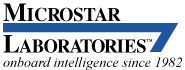## Knowledge Base: Processing

• See the list of other topics for category Processing

Other topic categories:

Q10062 Overflowing the limits of data ranges

Tags: Help, DAP, DAPL, processing, range limits, overflow, saturation, wrapping

Applies to: All DAP and xDAP products, all DAPL versions

What are the range limits for data captured by a Data Acquisition Processor? What happens if I accidentally overflow these range limits?

The fundamental limitations on data ranges arise from the implementations in the processor hardware. The processor implements the following data types that the DAPL system uses directly:

• `WORD` - 16-bit two's complement signed fixed point
• `LONG` - 32-bit two's complement signed fixed point
• `FLOAT` - 32-bit IEEE Standard 754 floating point arithmetic
• `DOUBLE` - 64-bit IEEE Standard 754 floating point arithmetic

Floating point limits rarely affect DAP users except in rare cases with ill-conditioned algorithms, perverse data scaling, and severe loss of significance in computations. You can look up the limits in the standards, or in typical C-language compiler "standard library" header files.

16-bit fixed point is a natural fit for most analog-to-digital converter devices, supporting a representable numeric range of -32768 (binary 0x8000) to +32767 (binary 0x7FFF). Since one value is reserved for 0 at center range, and the total number of converter codes is even, this results in the peculiar asymmetry between positive and negative. On DAP systems, the hardware converter ranges are aligned to the most-significant bits, and least significant bits are padded. Consequently, for converters with less than 16 bit resolution, lower-order bits of samples will always appear as zero, and samples don't reach the extreme limit of the positive numerical range. Sampling can never produce overflow conditions.

32-bit fixed point has a range -2147483648 to +2147483647. It is much harder to reach these limits, but it is possible to do so when performing calculations or when counting samples on very fast configurations that run for an extended period of time.

It is mostly when doing numerical computations with short fixed point numbers that you that you need to think about range limits. Processor overflow faults are disabled in the DAPL system – it would not be acceptable for ordinary computations to bring the processor to a virtual halt with numerical interrupt events. With overflow faults disabled, the default behavior in response to a range overflow, due to loss of a carry bit, is an apparent wraparound from very large numbers of one sign to very large numbers of the opposite sign. The wrapping behaviors are used to advantage for internal processing of trigger events and high-rate counter/timer monitoring. However, for purposes of numerical computations, the DAPL system suppresses the "wraparound" behaviors and enforces "saturating arithmetic." Thus, if you add a positive offset to a positive sampled value, and the result exceeds the numerical range limit, the result will be a maximum positive number, not an unexpected large-negative number. Processing in your custom-developed commands is not restricted in this manner, and you can impose any behavior that you want there.

The most common place to encounter saturation effects is in DAPL expression tasks. For example, supposed you evaluate the following expression task with 16-bit fixed point numbers:

`    POWER  =   (Pipe1*Pipe1) * 9995/10000 `

All of the terms are fixed point, so this computation is performed in 32-bit arithmetic. The first term `(Pipe1*Pipe1)` can never overflow, but the next computation produces the intermediate result `Pipe1*Pipe1*9995`. This could easily exceed the 32-bit numerical range – and the result is quietly saturated. This can lead to an unexpected final result.

You can avoid range limit problems like this in various ways, such as forcing the arithmetic to use floating point intermediate values:

`    POWER  =   (Pipe1*Pipe1) * 9995.0/10000.0 `

or you could re-order computations to preserve the significant bits at the expense of some loss of precision in the lower bits.

`    POWER  =   (Pipe1*Pipe1)/10000 * 9995 `

L-----

The DAPL 3000 Reference Manual covers number representations in more detail in the Voltages and Number Representation chapter.